/

### Function Description

Returns the solution,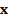, to a matrix equation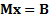given as inputs the LU decomposition and the corresponding LU index and permutation sign of the square (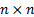) matrix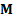(see MnMatLUDecomposition and MnMatLUIndexAndPerm) and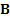is the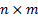matrix to solve for. The output is anmatrix.

Contents | Prev | Next